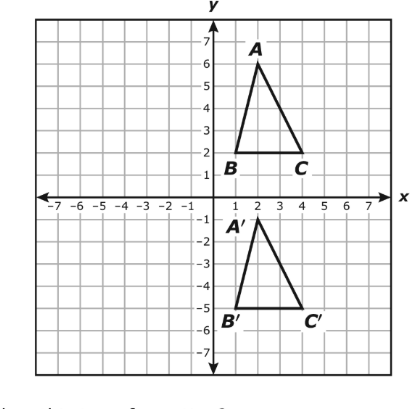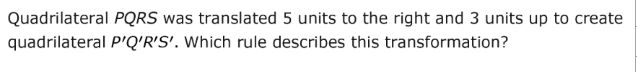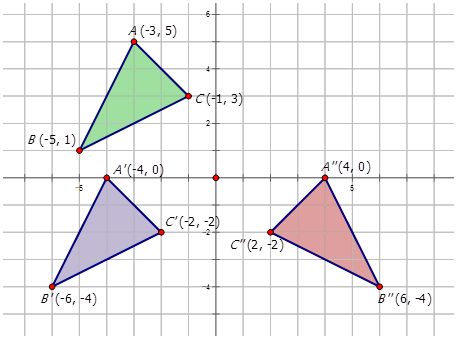Translations
Rotations
Reflections
Dilations
Composite Transformations
100

What is the definition of a Translation?

Change in location or slide

100

What is the definition of a Rotation?

Spin

100

What is the definition of a Reflection?

Flip, mirror image

100

What is the definition of a Dilation?

The image is getting bigger or smaller
100

Find the new coordinate of T(2,5) after a translation of (x-1,y+2) AND a reflection over the x-axis

T'(1,-7)

200

Find the coordinates of M(8,3) after a translation of (x-2,y+3)

M'(6,6)

200

Write the coordinate of R (-2,3) after a rotation of 90 degrees clockwise

(3,2)

200

Does this show a reflection over the x-axis or y-axis?

y-axis

200

What is the academic vocabulary for when an image is getting bigger? What is the academic vocabulary for when an image is getting smaller?

Bigger- Enlargement

Smaller- Reduction

200

Find the new coordinate of G(-3,7) after a translation of (x+3,y-4) AND a reflection over the y-axis

G'(0,3)

300

Write the algebraic expression of this transformation(x,y)->(x,y-7)

300

Write the coordinates of M(2,4) 90 degree counterclockwise rotation

M'(-4,2)

300

Find the coordinate of R after a reflection in the x-axis

R(-3,-6)

R'(-3,6)

300

Find the new coordinates after a dilation with scale factor k=3

M(5,12)

M(15,36)

300

Which transformations took place?Rotation  90 counterclockwise

Reflection of x-axis

400(x,y)->(x+5,x+3)

400

The rule (x,y)->(-y,x) is used. What is happening?

C 270, or CC 90

400

The image is using the rule (x,y)->(x,-y). What is happening?

Reflection over the x axis

400

Write the new coordinates after a dilation with scale factor k=1/2

T(-2,10)

T(-1,5)

400

Find the new coordinate of M(7,4)  after a translation of (x-2,y-2) AND a rotation of 90 degrees clockwise

M'(5,2)

M"(2,-5)

500

Write the coordinates of EFG after a translation of

(x-3,y+10)

E(8,2)

F(-2,-4)

G(-1,0)

E(5,12)

F(-5,6)

G(-4,10)

500

Find the coordinates after a 270 counterclockwise rotation

W(3,2)

S(5,7)

R(-1,0)

T(-2,-3)

W(2,-3)

S(7,-5)

R(0,1)

T(-3,2)

500

Find the coordinates of ABC after a reflection over the y-axis

A (2,4)

B (-3,-1)

C(-5,4)

A (-2,4)

B (3,-1)

C(5,4)

500

Find the scale factor of this dilation

R(4,7)---->R'(16,28)

k=4

500

What transformations took place?Translation rule (x-1,y-5) and reflection of y-axis

Click to zoom